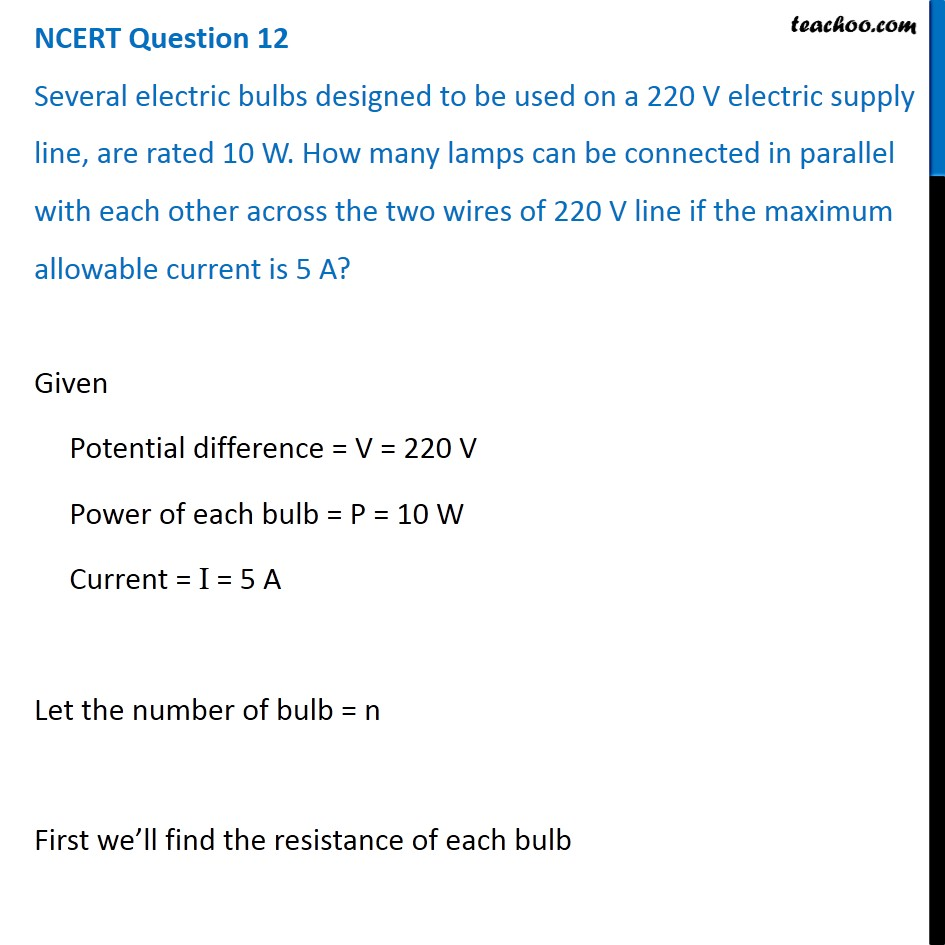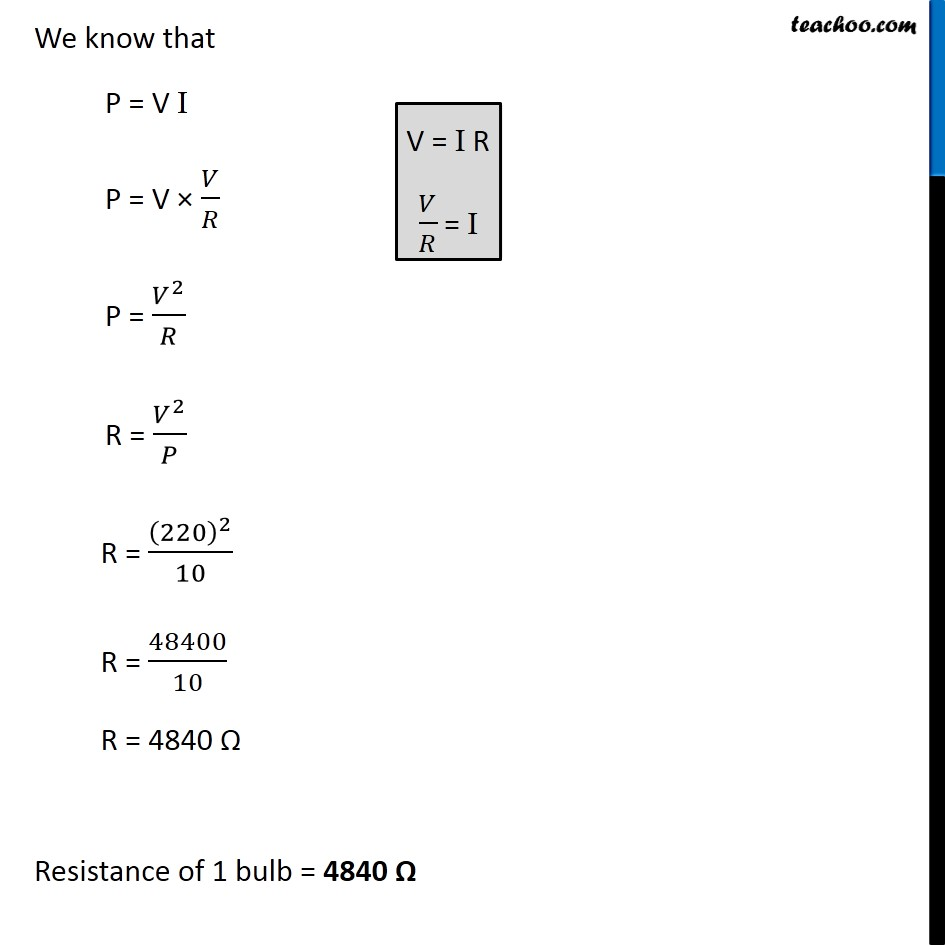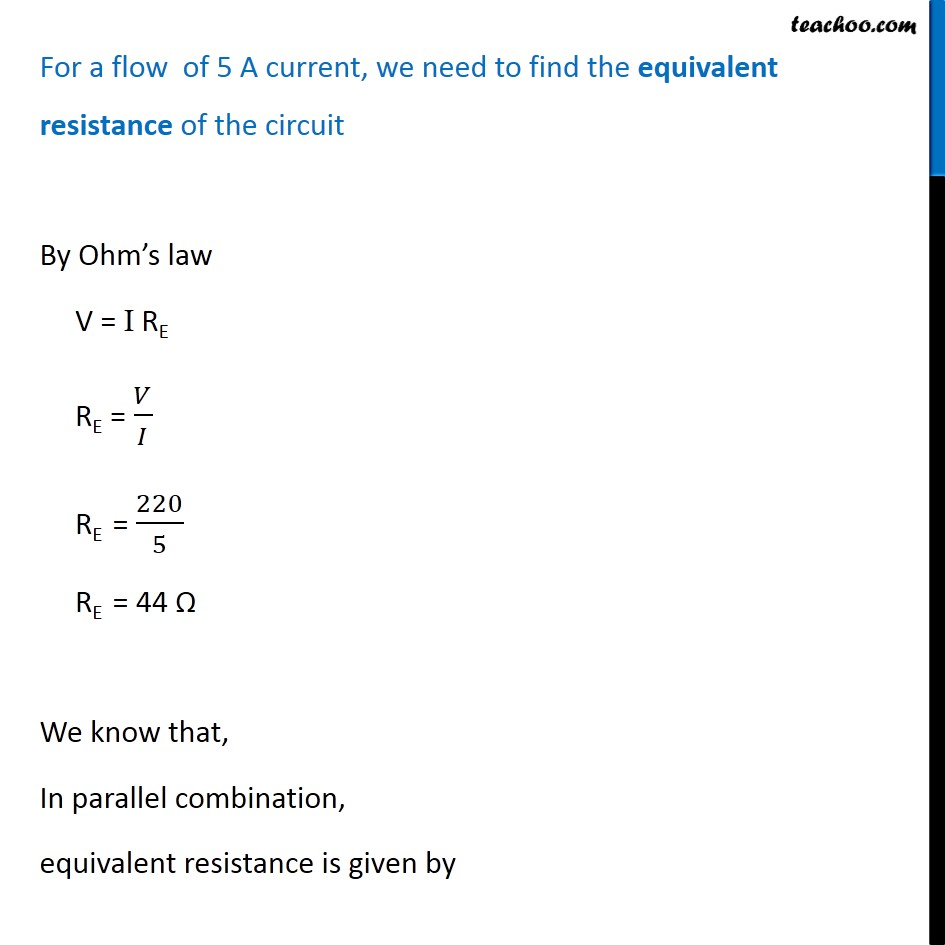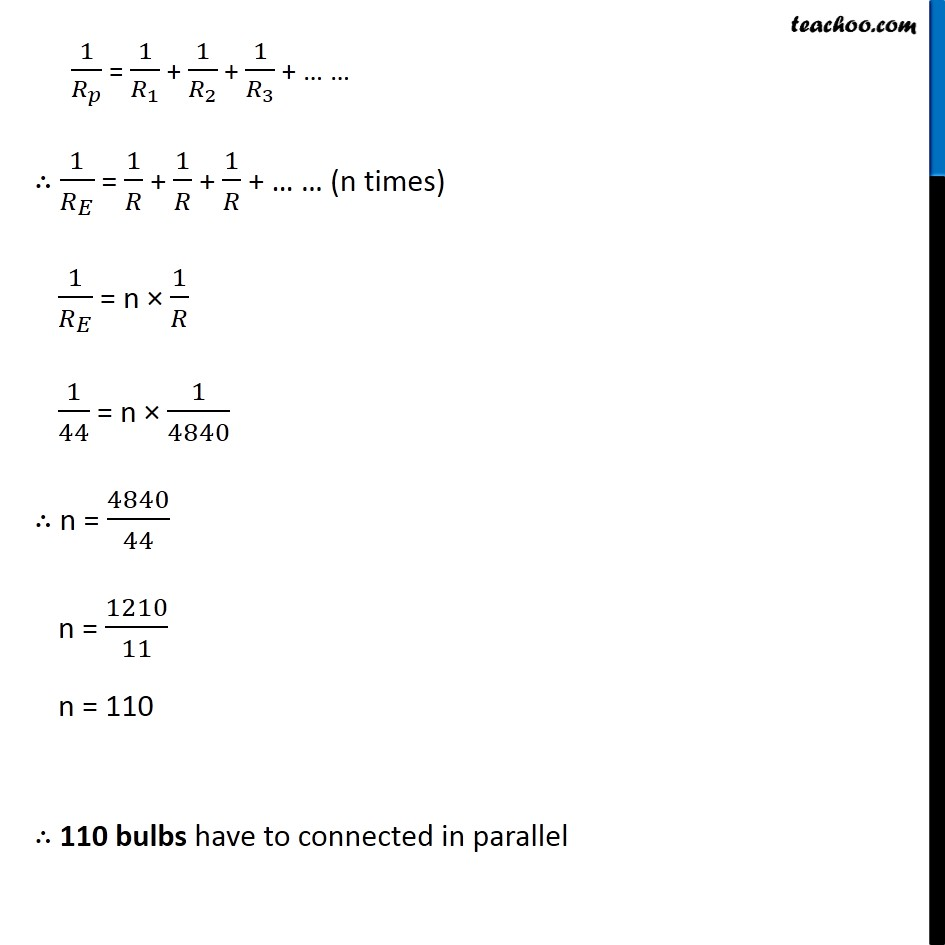NCERT Questions

Class 10
Chapter 12 Class 10 - Electricity

## Several electric bulbs designed to be used on a 220 V electric supply line, are rated 10 W. How many lamps can be connected in parallel with each other across the two wires of 220 V line if the maximum allowable current is 5 A?Learn in your speed, with individual attention - Teachoo Maths 1-on-1 Class

### Transcript

NCERT Question 12 Several electric bulbs designed to be used on a 220 V electric supply line, are rated 10 W. How many lamps can be connected in parallel with each other across the two wires of 220 V line if the maximum allowable current is 5 A? Given Potential difference = V = 220 V Power of each bulb = P = 10 W Current = I = 5 A Let the number of bulb = n First we’ll find the resistance of each bulb We know that P = V I P = V × 𝑉/𝑅 P = 𝑉^2/𝑅 R = 𝑉^2/𝑃 R = (220)^2/10 R = 48400/10 R = 4840 Ω Resistance of 1 bulb = 4840 Ω V = I R 𝑉/𝑅 = I For a flow of 5 A current, we need to find the equivalent resistance of the circuit By Ohm’s law V = I RE RE = 𝑉/𝐼 RE = 220/5 RE = 44 Ω We know that, In parallel combination, equivalent resistance is given by 1/𝑅_𝑝 = 1/𝑅_1 + 1/𝑅_2 + 1/𝑅_3 + … … ∴ 1/𝑅_𝐸 = 1/𝑅 + 1/𝑅 + 1/𝑅 + … … (n times) 1/𝑅_𝐸 = n × 1/𝑅 1/44 = n × 1/4840 ∴ n = 4840/44 n = 1210/11 n = 110 ∴ 110 bulbs have to connected in parallel